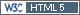daryazyatina
Junior

Ukraine,
2017-08-03 09:34

Posting: # 17646
Views: 13,108

## sample size in bioequivalence studies [Power / Sample Size]

Hi, guys!

Today I have a new question related to the the sample size formula for average bioequivalence.

Before now I сalculated the sample size for ABE by formula: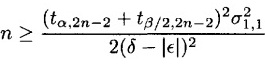(described in the book Shein-Chung Chow, Jun Shao and Hansheng Wang "Sample size calculations in clinical research" Copyright © 2003 by Marcel Dekker (Chapter 10. Bioequivalence Testing) http://www.crcnetbase.com/doi/book/10.1201/9780203911341)

I decided to try to calculate the sample size in R with package "PowerTOST" and function `sampleN.TOST()`

Comparing the results of the calculation in R and calculation with formula Shein-Chung Chow, Jun Shao and Hansheng Wang, I realized that they are different. I looked at the articles and books that this function refers to, and understand that different formulas are used.

On this basis, I had a question. What formula should I use to calculate the sample size for the ABE using two one-sided tests?
BE-proff
Senior

Russia,
2017-08-03 09:55

@ daryazyatina
Posting: # 17647
Views: 12,241

## sample size in bioequivalence studies

Hi darya,

Results must be different because PowerTOST uses iterative method for calculation.

AFAIK, PowerTOST is used worldwide and no claims from regulators have been received.
daryazyatina
Junior

Ukraine,
2017-08-03 10:08

@ BE-proff
Posting: # 17648
Views: 12,216

## sample size in bioequivalence studies

Hi, BE-proff. Thank you for your answer.

» Results must be different because PowerTOST uses iterative method for calculation.

Have you looked at the formula what I used? There is also used an iterative method.

» AFAIK, PowerTOST is used worldwide and no claims from regulators have been received.

It's good. But I asked another question.
I used formula from book:

» » Shein-Chung Chow, Jun Shao and Hansheng Wang "Sample size calculations in clinical research" Copyright © 2003 by Marcel Dekker (Chapter 10. Bioequivalence Testing)

and also any claims from regulators have not been received.
ElMaestro
Hero

Denmark,
2017-08-03 10:17

@ daryazyatina
Posting: # 17649
Views: 12,240

## sample size in bioequivalence studies

Hi Daryazyatina,

» I decided to try to calculate the sample size in R with package "PowerTOST" and function `sampleN.TOST()`

Good choice» Comparing the results of the calculation in R and calculation with formula Shein-Chung Chow, Jun Shao and Hansheng Wang, I realized that they are different. I looked at the articles and books that this function refers to, and understand that different formulas are used.

Can you tell what your design is and which values you want to plug in for the calculation? If the difference is 2 or 4 subjects, then so be it, subtle differences in approximation may account for that. If the difference is 46 or something then I'd wonder, too. I am sure there is an explanation and that your confidence in the powerTOST package can easily be restored. Apart from that you are of course right if you intended to hint that the author of the power.TOST family of R functions is a dubious character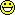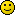``` if (3) 4 x=c("Foo", "Bar") b=data.frame(x) typeof(b[,1]) ##aha, integer? b[,1]+1 ##then let me add 1 ```

Best regards,
ElMaestro

"(...) targeted cancer therapies will benefit fewer than 2 percent of the cancer patients they’re aimed at. That reality is often lost on consumers, who are being fed a steady diet of winning anecdotes about miracle cures." New York Times (ed.), June 9, 2018.
daryazyatina
Junior

Ukraine,
2017-08-03 10:58

@ ElMaestro
Posting: # 17650
Views: 12,287

## sample size in bioequivalence studies

Hi ElMaestro,

» Good choiceThank you

» Can you tell what your design is and which values you want to plug in for the calculation? If the difference is 2 or 4 subjects, then so be it, subtle differences in approximation may account for that. If the difference is 46 or something then I'd wonder, too. I am sure there is an explanation and that your confidence in the powerTOST package can easily be restored.

For comparison, I use all the standard properties. Design 2x2, confidence intervals 0.8 - 1.25, power 0.8, alpha 0.05.
The only thing about what I'm not sure is CV. Because in formula that I used this is intra-subject variability, but in PowerTOST() this is coefficient of variation as ratio. In calculations in both cases I used СV - 0.3.

And this is results from `sampleN.TOST()`
`sampleN.TOST(logscale = TRUE, CV = 0.3, details = TRUE)`
```+++++++++++ Equivalence test - TOST +++++++++++             Sample size estimation ----------------------------------------------- Study design:  2x2 crossover log-transformed data (multiplicative model) alpha = 0.05, target power = 0.8 BE margins = 0.8 ... 1.25 True ratio = 0.95,  CV = 0.3 Sample size (total)  n     power 40   0.815845 ```
The sample size by the formula I used:

n=28

» Apart from that you are of course right if you intended to hint that the author of the power.TOST family of R functions is a dubious character.DavidManteigas
Regular

Portugal,
2017-08-03 12:40

@ daryazyatina
Posting: # 17651
Views: 12,293

## sample size in bioequivalence studies

Hi daryazyatina,

For some reason, I can't see the image with the formula that you put on the first post.

» For comparison, I use all the standard properties. Design 2x2, confidence intervals 0.8 - 1.25, power 0.8, alpha 0.05.
» The only thing about what I'm not sure is CV. Because in formula that I used this is intra-subject variability, but in PowerTOST() this is coefficient of variation as ratio. In calculations in both cases I used СV - 0.3.

Within subject standard deviation and within subject CV are different parameters. Nevertheless, I think that this is not the only reason for such a big difference.

There is a sentence in one of the articles that is quoted on SampleNTOST formula that may clarify this issue:

"This formula is less conservative than Formula (5), but it may result in a lower actual power than the required. For example, when α = 0.05, σ = 0.3, Δ = 0.2, θ = 0.01 and a required power = 0.80, the sample size from Formula (6) [Formula from Chow] is 17 per sequence, but the actual power obtained by this sample size is only 0.69."

So by reading this, I am not sure if Chow formula might be appropriate to calculate sample size for BABE trials. I have just quickly read the article, so I may not be doing a proper analysis. Perhaps dlabes might clarify this, since he is the master that we all should thank for the amazing PowerTOST packagedaryazyatina
Junior

Ukraine,
2017-08-03 13:46

@ DavidManteigas
Posting: # 17652
Views: 12,129

## sample size in bioequivalence studies

Hi, DavidManteigas

» For some reason, I can't see the image with the formula that you put on the first post.

Look again at the picture with formula. At first it was not visible, now you can see it.

» Within subject standard deviation and within subject CV are different parameters. Nevertheless, I think that this is not the only reason for such a big difference.

I did not write anything about the standard deviation.

» "This formula is less conservative than Formula (5), but it may result in a lower actual power than the required. For example, when α = 0.05, σ = 0.3, Δ = 0.2, θ = 0.01 and a required power = 0.80, the sample size from Formula (6) [Formula from Chow] is 17 per sequence, but the actual power obtained by this sample size is only 0.69."

If you looked at the formula I use, you would see in the article that this is another formula Chow. And that's why I do not understand what formula should be used.

» So by reading this, I am not sure if Chow formula might be appropriate to calculate sample size for BABE trials. I have just quickly read the article, so I may not be doing a proper analysis. Perhaps dlabes might clarify this, since he is the master that we all should thank for the amazing PowerTOST packageI want to understand how to do it right, just this.Helmut
HeroVienna, Austria,
2017-08-03 15:37

@ DavidManteigas
Posting: # 17653
Views: 12,284

## Don’t use the formula by Chow, Shao, Wang!

Hi David & Darya,

» For some reason, I can't see the image with the formula that you put on the first post.

I uploaded a copy. Should be visible by now.

» » The only thing about what I'm not sure is CV. Because in formula that I used this is intra-subject variability, but in PowerTOST() this is coefficient of variation as ratio. In calculations in both cases I used СV - 0.3.
»
» Within subject standard deviation and within subject CV are different parameters. Nevertheless, I think that this is not the only reason for such a big difference.

Chow used the standard deviation, whilst in `Power.TOST` the CV (fraction, not in %!) is used. However, no big difference since CV = √ – 1 and the other way ’round s = √log(CV² + 1). In `Power.TOST` for convenience you can use `se2CV(foo)` for the former and `CV2se(foo)` for the latter.

» There is a sentence in one of the articles that is quoted on SampleNTOST formula that may clarify this issue: […]
» So by reading this, I am not sure if Chow formula might be appropriate to calculate sample size for BABE trials.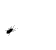I think it’s crap. The formula Darya posted (10.2.6) of p.259 of the book gives the impression that n is the total sample size. The text continues with:

Since the above equations do not have an explicit solution, for convenience, for a 2 × 2 crossover design, the total sample size needed to achieve a power of 80% or 90% at 5% level of significance with various combinations of ε and δ is given in Table 10.2.1.

However, in the example which follows on p.260 we read:

By referring to Table 10.2.1, a total of 24 subjects per sequence is needed in order to achieve an 80% power at the 5% level of significance.

(my emphases)

» […] Perhaps dlabes might clarify this, since he is the master that we all should thank for the amazing PowerTOST packageDetlew is on vacation. Some clarifications:

Zhang1
(5) where n = sample size per sequence(9) with a correction term c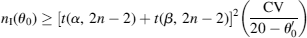Hauschke et al.2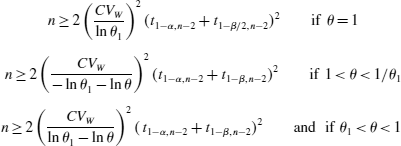Chow & Liu (5.4.10)3Using the formulas of Zhang or Chow & Liu, you get the sample size / sequence. To obtain the total, multiply by 2, which is already done it the right-hand side of Hauschke’s formulas.

Comparison with the references:
Table 2, untransformed data, 90% Power, Δ 0.2, σ 0.3, ∕θ 0.05 (p.5371): 37 / sequence

```library(PowerTOST) sampleN.TOST(CV=se2CV(0.3), theta0=0.05, theta1=-0.2, theta2=0.2,              logscale=FALSE, targetpower=0.90,              print=FALSE)[["Sample size"]]/2  37```Table 5.4.1, untransformed data, 80% Power, Δ 0.2μR, α 0.05 (p.1583): 52

```library(PowerTOST) sampleN.TOST(CV=0.30, theta0=0.05, theta1=-0.2, theta2=0.2,              logscale=FALSE, targetpower=0.80,              print=FALSE)[["Sample size"]]  52```Table 5.1, log-transformed data, 80% Power, (θ1, 1∕θ1) = (0.80, 1.25), θ 0.95, α 0.05 (p.1132): 40

```library(PowerTOST) sampleN.TOST(CV=0.30, theta0=0.95, theta1=0.8, theta2=1.25,              targetpower=0.80,              print=FALSE)[["Sample size"]]  40```Stop using the formula given by Chow, Shao, Wang! Sample sizes are way too small – which compromises power. If you used it in the past for 80% power – if all assumptions (CV, θ0) turned out to be “correct” – substantially more than 20% of studies should have failed. If not, you were lucky!

1. Zhang P. A Simple Formula for Sample Size Calculation in Equivalence Studies. J Biopharm Stat. 2003;13(3):529-38. doi:10.1081/BIP-120022772
2. Hauschke D, Steinijans V, Pigeot I. Bioequivalence Studies in Drug Development. Chichester: John Wiley; 2007. p.116. doi:10.1002/9780470094778
3. Chow S-C Liu J-p. Design and Analysis of Bioavailability and Bioequivalence Studies.
Boca Raton: Chapman & Hall/CRC Press; 3rd ed. 2009. p.157. doi:10.1201/9781420011678

Cheers,
Helmut SchützThe quality of responses received is directly proportional to the quality of the question asked. ☼
Science Quotes
daryazyatina
Junior

Ukraine,
2017-08-03 15:52

@ Helmut
Posting: # 17654
Views: 12,066

## Don’t use the formula by Chow, Shao, Wang!

Hi, Helmut

» Stop using the formula given by Chow, Shao, Wang! Sample sizes are way too small – which compromises power. If you used it in the past for 80% power – if all assumptions (CV, θ0) turned out to be “correct” – substantially more than 20% of studies should have failed. If not, you were lucky!

Thank you for such a comprehensive answer.
DavidManteigas
Regular

Portugal,
2017-08-03 16:12

@ Helmut
Posting: # 17657
Views: 12,103

## Don’t use the formula by Chow, Shao, Wang!

Thank you for the clarification Helmut, very helpful as always.

In the example you've mentioned on page 260 there is also another mistake, since z0.10 is 1.28 and not 0.84, so according to the reduced formula, 28 subjects per sequence would be necessary and not the reported 21. Still, significantly lower than the sample obtained with sampleNTOST.
Helmut
HeroVienna, Austria,
2017-08-03 16:33

@ DavidManteigas
Posting: # 17658
Views: 12,220

## Don’t use the formula by Chow, Shao, Wang!

Hi David,

» […] on page 260 there is also another mistake, since z0.10 is 1.28 and not 0.84, so according to the reduced formula, 28 subjects per sequence would be necessary and not the reported 21.

Yep, I noticed that as well.BTW, z0.05 is 1.64 and not 1.96. In all its “beauty”:

``` delta    <- log(1.25) epsilon  <- 0.05 sigma1.1 <- 0.40 z.05     <- qnorm(0.05, lower.tail=FALSE) z.10     <- qnorm(0.10, lower.tail=FALSE) (z.05+z.10)^2*sigma1.1^2/(2*(delta-epsilon)^2)  22.85316```

Of course, `PowerTOST` contains the large sample approximation based on z as well (by an internal and hence, undocumented) function.

```library(PowerTOST) CV <- seq(0.15, 0.8, 0.025) n  <- data.frame(CV=CV, exact=rep(NA, length(CV)),                  nc.t=rep(NA, length(CV)),                  shifted.t=rep(NA, length(CV)),                  normal=rep(NA, length(CV)),                  row.names=NULL) for (j in seq_along(CV)) {   # exact method (Owen's Q)   n[j, 2] <- sampleN.TOST(CV=CV[j], theta0=0.95, theta1=0.8,                           theta2=1.25, targetpower=0.8,                           method="exact", print=FALSE)[["Sample size"]]   # approximation by the noncentral t-distribution   n[j, 3] <- sampleN.TOST(CV=CV[j], theta0=0.95, theta1=0.8,                           theta2=1.25, targetpower=0.8,                           method="nct", print=FALSE)[["Sample size"]]   # approximation by the shifted central t-distribution   n[j, 4] <- sampleN.TOST(CV=CV[j], theta0=0.95, theta1=0.8,                           theta2=1.25, targetpower=0.8,                           method="shifted",                           print=FALSE)[["Sample size"]]   # (large sample) approximation by the normal distribution   n[j, 5] <- PowerTOST:::.sampleN0(se=CV2se(CV[j]), diffm=log(0.95),                                    ltheta1=log(0.8), ltheta2=log(1.25),                                    targetpower=0.8) } print(n, row.names=FALSE)```

Gives:

```    CV exact nc.t shifted.t normal  0.150    12   12        12     10  0.175    16   16        16     12  0.200    20   20        20     16  0.225    24   24        24     20  0.250    28   28        28     26  0.275    34   34        34     30  0.300    40   40        40     36  0.325    46   46        46     42  0.350    52   52        52     48  0.375    58   58        60     56  0.400    66   66        66     62  0.425    74   74        74     70  0.450    82   82        82     78  0.475    90   90        90     86  0.500    98   98        98     94  0.525   106  106       108    102  0.550   116  116       116    110  0.575   126  126       126    120  0.600   134  134       134    128  0.625   144  144       144    138  0.650   154  154       154    148  0.675   164  164       164    158  0.700   174  174       174    166  0.725   184  184       184    176  0.750   194  194       194    186  0.775   204  204       204    196  0.800   214  214       216    208```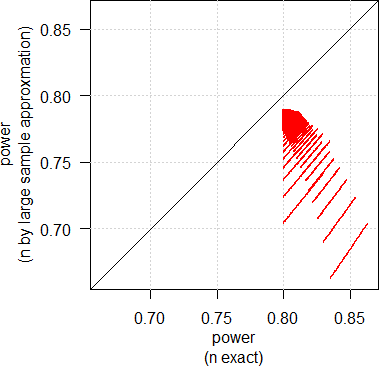The approximation by the noncentral t-distribution does an excellent job. That’s why we’ve set it as the default in package `Power2Stage` for speed reasons (~40 times faster than Owen’s Q).
The shifted central t is also good. Only in a few cases higher sample sizes. Conservative, no worries.
The large sample size approximation sucks. Always smaller sample sizes than with the other methods, power compromised – unless one dares to submit a study evaluated by z.Quoting from a conversation with an eminent regulator of the Iberian Peninsula:

“Frankly between z and t methods the difference is ridiculous when variability is not large and later a few subjects is added to compensate drop-outs. I do not see any problem in using z-method. I use it because it is very straight­forward in Excel and there is no need to have special software.”

Well, cough…

Brain-dead. 38.9 ℃ and rising, no AC in my office…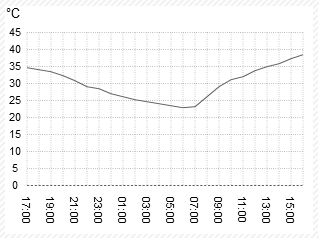Cheers,
Helmut SchützThe quality of responses received is directly proportional to the quality of the question asked. ☼
Science Quotes
d_labes
Hero

Berlin, Germany,
2017-08-16 16:01

@ Helmut
Posting: # 17694
Views: 11,578

## Don’t use the Book by Chow, Shao, Wang!

Hi all!

In addition to all what was said up to now:
Don’t use the Book by Chow, Shao, Wang!

It's full of errors, full of a terminology not compatible to other publications so that one has no chance to compare.
(F.i. what are epsilon, delta, sigma1.1 compared to our use of theta0, upper or lower BE limit, intra-subject CV. No one knows! To cite our ol'Sailor "garbage in, garbage out, It's that simple.")

Regards,

Detlew
Helmut
HeroVienna, Austria,
2017-08-03 16:04

@ daryazyatina
Posting: # 17655
Views: 12,086

## compromised power

Hi Darya,

» The sample size by the formula I used:
» n=28

To keep everything equal you should convert the standard deviation to the CV. Hence,

```library(PowerTOST) sampleN.TOST(CV=se2CV(0.3)) +++++++++++ Equivalence test - TOST +++++++++++             Sample size estimation ----------------------------------------------- Study design:  2x2 crossover log-transformed data (multiplicative model) alpha = 0.05, target power = 0.8 BE margins = 0.8 ... 1.25 True ratio = 0.95,  CV = 0.3068783 Sample size (total)  n     power 42   0.818541```

Of course higher than the 28 by the dubious formula. As David mentioned above (quoting Zhang’s paper) power is compromised. Let’s check how much:

```power.TOST(CV=se2CV(0.3), n=28)  0.6250166```

Oops!

Cheers,
Helmut SchützThe quality of responses received is directly proportional to the quality of the question asked. ☼
Science Quotes
daryazyatina
Junior

Ukraine,
2017-08-04 08:15

@ Helmut
Posting: # 17663
Views: 11,957

## compromised power

Hi Helmut,

» To keep everything equal you should convert the standard deviation to the CV. Hence,
» `se2CV(0.3)`

You are wrote about converting the standard deviation to СV, but use the formula to convert the standard error to СV. I thought that the standard deviation and standard error are different parameters. Maybe I'm wrong.
Helmut
HeroVienna, Austria,
2017-08-04 12:41

@ daryazyatina
Posting: # 17664
Views: 11,972

## terminology

Hi Darya,

» You are wrote about converting the standard deviation to СV, but use the formula to convert the standard error to СV. I thought that the standard deviation and standard error are different parameters.

Like in this thread it is a question of terminology; see this thread for details.
In `PowerTOST` type `?se2CV` to get the formulas for conversion. If you want a second opinion:1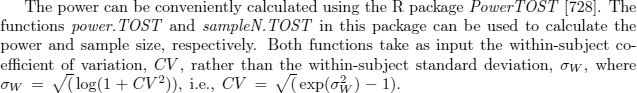Nitpick: Chaos everywhere. We have only sw – which is the estimate of the unknown σw
BTW, when it comes to reference-scaling, both the EMA and the FDA correctly use swR. Since scaling is based on the unknown parameters, we might observe an inflation of the type I error.2

1. Patterson S, Jones B. Bioequivalence and Statistics in Clinical Pharmacology. Boca Raton: Chapman & Hall / CRC; 2nd ed. 2016: p.47.
2. Labes D, Schütz H. Inflation of Type I Error in the Evaluation of Scaled Average Bioequivalence, and a Method for its Control. Pharm Res. 2016;33(11):2805–14. doi:10.1007/s11095-016-2006-1.

Cheers,
Helmut SchützThe quality of responses received is directly proportional to the quality of the question asked. ☼
Science Quotes
daryazyatina
Junior

Ukraine,
2017-08-16 10:12

@ Helmut
Posting: # 17689
Views: 11,635

## terminology

Hi Helmut

» Like in this thread it is a question of terminology; see this thread for details.

Some articles describe the difference between a standard deviation and a standard error. How to understand such articles? for example

The terms “standard error” and “standard deviation” are often confused. The contrast between these two terms reflects the important distinction between data description and inference, one that all researchers should appreciate.1
1. Douglas G Altman, J Martin Bland. Standard deviations and standard errors.
doi:10.1136/bmj.331.7521.903
2. David L Streiner, PhD. Maintaining Standards: Differences between the Standard Deviation and Standard Error, and When to Use Each.
http://ww1.cpa-apc.org/Publications/Archives/PDF/1996/Oct/strein2.pdf
Helmut
HeroVienna, Austria,
2017-08-16 18:54

@ daryazyatina
Posting: # 17695
Views: 11,642

## terminology

Hi Darya,

» Some articles describe the difference between a standard deviation and a standard error.

THX for the second one! Was great fun to read.

» How to understand such articles?

Duno. Perfect description. Read them again.Cheers,
Helmut SchützThe quality of responses received is directly proportional to the quality of the question asked. ☼
Science Quotes
balinskyi
Junior

Ukraine,
2018-06-03 12:58

@ daryazyatina
Posting: # 18844
Views: 7,511

## sample size in bioequivalence studies

Hello everyone,

I should note, that this formula was published in Ukrainian Pharmacopoeia 1st edition (obsolete since 2016) with reference to Liu and Chow 2008 book. Now, while planning a study, the sponsor has applied the formula (referring to Pharmacopoeia) and consequently argue, that my estimate for the sample size (calculated with PowerTOST) is unnecessarily high. So in my search for an answer I have found this thread. Thank you everyone for the answers!
Bioequivalence and Bioavailability Forum |  Admin contact
19,271 posts in 4,099 threads, 1,315 registered users;
online 23 (1 registered, 22 guests [including 11 identified bots]).

It’s easy to lie with statistics;
it is easier to lie without them.    Frederick Mosteller

The BIOEQUIVALENCE / BIOAVAILABILITY FORUM is hosted byIng. Helmut Schütz